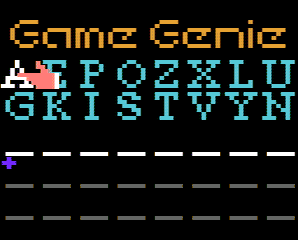ddaa's blog
Write-ups for CTF.
ddaa's blog

# GiTs 2014 Trivia 150 lugkist

GVZSNG AXZIOG YNAISG ASAIUG ....`d w P m a ? d i r c i e l m y w n c a a v p a h s i k v t s n h t e n c e h o B g s a o r e d e . y o e`

`P o w e r o v e r w h e l m i n g ? B a c k i n m y d a y c h e a t s d i d n o t h a v e s p a c e s .`

flag: `Power overwhelming? Back in my day cheats did not have spaces.`